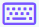:

Easy
Medium
Hard

## Derivative calculator with steps

Derivative calculator (A.K.A differentiation calculator) is used to determine the rate of change of the given function with respect to its independent variable. The function can be constant, linear, polynomial, quadric polynomial, etc.

The differential calculator will recognize the function and calculate its derivative. There are three kinds of differential.

This derivative solver evaluates the explicit differentiation of any function with just one click.

## How does this differentiation calculator work?

To solve the problems of explicit differentiation, follow the steps below.

• Enter the constant, linear, or polynomial function into the input box.
• Select the independent variable like x, y, z, u, v, t, or w.
• Use the keypad iconfor writing the arithmetic and other symbols like √, +, -, e, etc.
• Write the number of derivatives e.g, 1 for the first derivative or 2 for the second derivative.
• Click the calculate button below the input box to get the results.
• Press the reset button to enter another function.

## What is derivative?

The derivative is the rate of change of the function with respect to its variable. Derivatives are fundamental to the solution of problems in differential equations and calculus.

The inverse process of differentiation is known as integral.

### Rules of differentiation

Here are some basic rules of differentiation are mentioned below.

 Names Rules Constant rule $$\frac{d}{dx}\left(c\right)=0$$ Power rule $$\frac{d}{dx}\left(u\right)^n=nu^{n-1}$$ Sum rule $$\frac{d}{dx}\left(u\left(x\right)+v\left(x\right)\right)=\frac{d}{dx}\left(u\left(x\right)\right)+\frac{d}{dx}\left(v\left(x\right)\right)$$ Difference rule $$\frac{d}{dx}\left(u\left(x\right)-v\left(x\right)\right)=\frac{d}{dx}\left(u\left(x\right)\right)-\frac{d}{dx}\left(v\left(x\right)\right)$$ Product rule $$\frac{d}{dx}\left(u\left(x\right)\cdot v\left(x\right)\right)=v\left(x\right)\frac{d}{dx}\left(u\left(x\right)\right)+u\left(x\right)\frac{d}{dx}\left(v\left(x\right)\right)$$ Quotient rule $$\frac{d}{dx}\left(\frac{u\left(x\right)}{v\left(x\right)}\right)=\frac{v\left(x\right)\frac{d}{dx}\left(u\left(x\right)\right)-u\left(x\right)\frac{d}{dx}\left(v\left(x\right)\right)}{\left(v\left(x\right)\right)^2}$$

## How to calculate derivatives?

Below are some examples solved by using our d/dx calculator.

Example

Calculate the derivative of $$x^2+3x$$

Solution

Step 1: Apply the derivative notation in the given expression.

$$\frac{d}{dx}\left(x^2+3x\right)$$

Step 2: To solve the above function, apply the sum and the power rule.

$$\frac{d}{dx}\left(x^2+3x\right)$$ = $$\frac{d}{dx}\left(x^2\right)$$ + $$\frac{d}{dx}\left(3x\right)$$
$$\frac{d}{dx}\left(x^2+3x\right)$$= $$2x^{2-1}+3x^{1-1}$$
$$\frac{d}{dx}\left(x^2+3x\right)$$ = $$2x^1+3x^0$$
$$\frac{d}{dx}\left(x^2+3x\right)$$ = $$2x+3\left(1\right)$$
$$\frac{d}{dx}\left(x^2+3x\right)$$= $$2x+3$$

## Table of derivative of functions

Here are some well-known examples of differentiation solved by our differential calculator.

 Question Answer Derivative of e^x e^x Derivative of 2 0 Derivative of x 1 Derivative of 2^x 2^x ln2 Derivative of 1/x -1/x^2 Derivative of a^x ln(a)a^x Derivative of ln(x) 1/x Derivative of 2*1 0 Derivative of sinx cosx Derivative of cosx -sinx Derivative of tanx sec^2x Derivative of secx tanx secx Derivative of sin(3x) 3cos3x Derivative of sin2x 2cos2x Derivative of sin^2x 2sinx cosx Derivative of cos^3x -3sinx cos^2x Derivative of sin(3x+1) 3cos(3x+1) Derivative of sin^4x 4sin^3x cosx Derivative of cotx -csc^2x Derivative of tan2x 2sec^2(2x) Derivative of sec^2x 2tanxsec^2x Derivative of 2x 2 Derivative of 1/sqrt(x) -1/2x^(3/2) Derivative of root x ½ x^ (-1/2) Derivative of 1/e^x -e^(-x) Derivative of 1/sinx -cot(x)csc(x) Derivative of 1/cosx tan(x)sec(x) Derivative of 1/(1+x^2) -2x/(1+x^2)^2

### References

Use android or iOS app of our limit calculator on your mobile